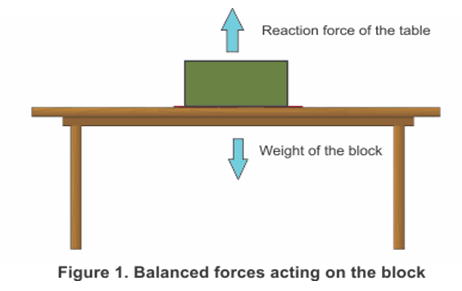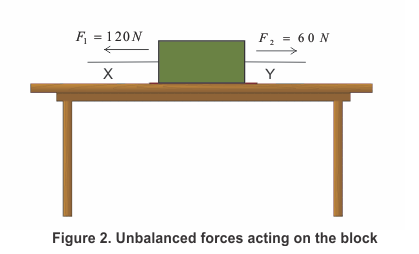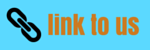# Force

Notes Assignments NCERT Solutions

## 1. Introduction

• While studying kinematics, we have already studied about the position, displacement and acceleration of a moving particle.
• Here in this chapter, we would take our understanding one step further to learn about origins of acceleration or force.
• Here we will specifically consider the cause behind the moving objects i.e. what causes the objects to move.
• Thus we will learn the theory of motion based on the ideas of mass and force and the laws connecting these physical concepts to the kinematics quantities.
• Concept of force is central to all of physics whether it is classical physics, nuclear physics, quantum physics or any other form of physics
• So what is force? When we push or pull anybody we are said to exert force on the body
• Push or pull applied on a body does not exactly define the force in general. We can define force as an influence causing a body at rest or moving with constant velocity to undergo acceleration
• There are many ways in which one body can exert force on another body. Few examples are given below
(a) Stretched springs exerts force on the bodies attached to its ends
(b) Compressed air in a container exerts force on the walls of the container
(c) Force can be used to deform a flexible object
• Force of gravitational attraction exerted by earth is a kind of force that acts on every physical body on the earth and is called the weight of the body

## 2. Balanced and Unbalanced forces

• There are two types of forces namely balanced forces and unbalanced forces

### (a) Balanced Forces

• If the resultant of all forces acting on a body is zero then the forces are called balanced forces.
• To understand this concept consider an object rests on a surface such as a block on the table as shown below in the figure 1. Weight of the block is balanced by the reaction force from the table. The table pushes up against the block.So the weight of a block lying on a table is balanced by the reaction force from the table top
• If the forces on an object are balanced (or if there are no forces acting on it) than the object that is not moving stays still and the object that is moving continues to move at the same speed and in the same direction.
• Although balanced forces cannot produce motion in a stationary body or stop a moving body but they can however change the shape of the body.

### (b) Unbalanced Forces

• If the resultant forces acting on a body is not zero the forces are called unbalanced forces.
• To understand this consider the figure 2 which shows a block on a horizontal table and two strings X and Y are tied to the two opposite faces of the block.• Let us now pull the block using this string in two different directions such that two opposite forces of different magnitudes acts on the block.
• Since two forces acting on the block are of different magnitude the block would begin to move in the direction of the greater force.
• Thus, the two forces acting on the block are not balanced and the unbalanced force acts in the direction the block moves.
• So unbalanced forces can move a stationary body and they can stop a moving body.
• The size of the overall force acting on an object is called the resultant force. If the forces are balanced, this is zero. In the example above, the resultant force is the difference between the two forces F1 and F2, which is 120 - 60 = 60 N.
• If all the forces acting on a body result in an unbalanced force, then the unbalanced force can accelerate the body. It means that a net force or resulting force acting on a body can either change the magnitude of its velocity or change the direction of its velocity.
• The force that opposes the relative motion between the surfaces of two objects in contact and acts along the surfaces in contact is called the force of friction or simply friction.